SSC CGL Previous Year Questions: Data Interpretation - 6

# SSC CGL Previous Year Questions: Data Interpretation - 6 - SSC CGL

Test Description

## 30 Questions MCQ Test SSC CGL (Tier - 1) - Previous Year Papers (Topic Wise) - SSC CGL Previous Year Questions: Data Interpretation - 6

SSC CGL Previous Year Questions: Data Interpretation - 6 for SSC CGL 2023 is part of SSC CGL (Tier - 1) - Previous Year Papers (Topic Wise) preparation. The SSC CGL Previous Year Questions: Data Interpretation - 6 questions and answers have been prepared according to the SSC CGL exam syllabus.The SSC CGL Previous Year Questions: Data Interpretation - 6 MCQs are made for SSC CGL 2023 Exam. Find important definitions, questions, notes, meanings, examples, exercises, MCQs and online tests for SSC CGL Previous Year Questions: Data Interpretation - 6 below.
Solutions of SSC CGL Previous Year Questions: Data Interpretation - 6 questions in English are available as part of our SSC CGL (Tier - 1) - Previous Year Papers (Topic Wise) for SSC CGL & SSC CGL Previous Year Questions: Data Interpretation - 6 solutions in Hindi for SSC CGL (Tier - 1) - Previous Year Papers (Topic Wise) course. Download more important topics, notes, lectures and mock test series for SSC CGL Exam by signing up for free. Attempt SSC CGL Previous Year Questions: Data Interpretation - 6 | 30 questions in 30 minutes | Mock test for SSC CGL preparation | Free important questions MCQ to study SSC CGL (Tier - 1) - Previous Year Papers (Topic Wise) for SSC CGL Exam | Download free PDF with solutions
 1 Crore+ students have signed up on EduRev. Have you?
SSC CGL Previous Year Questions: Data Interpretation - 6 - Question 1

### The following graph represents the maximum and minimum temperature recorded every day in a certain week. The day on which the difference between the maximum and minimum temperature was maximum is       (SSC Multitasking 2013)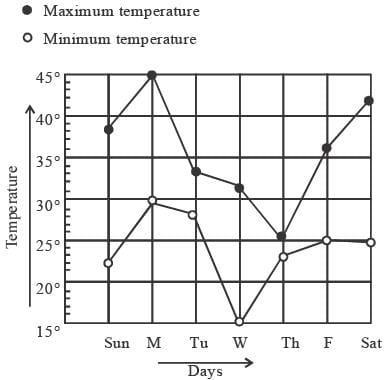Detailed Solution for SSC CGL Previous Year Questions: Data Interpretation - 6 - Question 1

According to graph,
Difference between the maximum and minimum temperature was maximum on Saturday.

SSC CGL Previous Year Questions: Data Interpretation - 6 - Question 2

### DIRECTIONS: The following pie-chart represents the profits earned by a certain company in seven consecutive years. Study the pie-chart carefully and answer the question.        (SSC CGL 2ndSit.2012)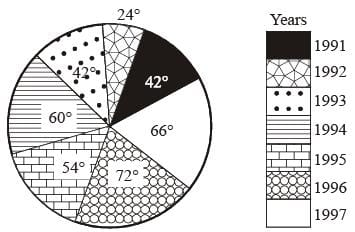Q. The year in which the profit is nearest to the average of the profits earned in all the given years is

Detailed Solution for SSC CGL Previous Year Questions: Data Interpretation - 6 - Question 2

Average profits earned in all the given years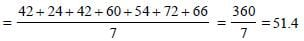This is approximately equal to the profit earned in the year 1995.

SSC CGL Previous Year Questions: Data Interpretation - 6 - Question 3

### DIRECTIONS: The following pie-chart represents the profits earned by a certain company in seven consecutive years. Study the pie-chart carefully and answer the question.        (SSC CGL 2ndSit.2012)Q. If x% of the total of profits earned in all the given years is same as the profit earned in the year 1994, then x is

Detailed Solution for SSC CGL Previous Year Questions: Data Interpretation - 6 - Question 3

Required value = (60/360) x 100 = 16(2/3)%

SSC CGL Previous Year Questions: Data Interpretation - 6 - Question 4

DIRECTIONS: The following pie-chart represents the profits earned by a certain company in seven consecutive years. Study the pie-chart carefully and answer the question.        (SSC CGL 2ndSit.2012)Q. If the expenditure in the year 1993 was 30% more than the expenditure in the year 1991, then the income in the year 1993 exceeds the income in the year 1991 by 30% of

Detailed Solution for SSC CGL Previous Year Questions: Data Interpretation - 6 - Question 4

According to graph, the expenditure in the year 1993 was 30% more than the expenditure in the year 1991, the income in the year 1993 exceeds the income in the year 1991 by 30% of the expenditure in the year 1991.

SSC CGL Previous Year Questions: Data Interpretation - 6 - Question 5

DIRECTIONS: Population of five adjacent areas of a town, in the year of 2010, are represented in the following Piechart. the ratio of the numbers of males to that of females in these areas are stated in the table below. The total of the population in all the five areas is 72 lakh. Study the Pie-chart and the table and then answer the questions.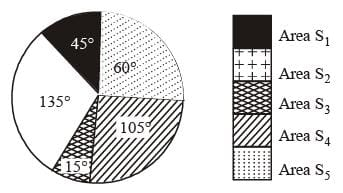Ratio of numbers of males (M) to females (F)       (SSC CGL 1st Sit. 2012)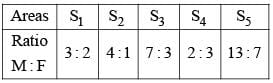Q. 12 lakh is the population of the area

Detailed Solution for SSC CGL Previous Year Questions: Data Interpretation - 6 - Question 5

∵ 72 lakhs ≡ 360°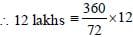60° = S5

SSC CGL Previous Year Questions: Data Interpretation - 6 - Question 6

DIRECTIONS: Population of five adjacent areas of a town, in the year of 2010, are represented in the following Piechart. the ratio of the numbers of males to that of females in these areas are stated in the table below. The total of the population in all the five areas is 72 lakh. Study the Pie-chart and the table and then answer the questions.Ratio of numbers of males (M) to females (F)       (SSC CGL 1st Sit. 2012)Q. The number of males in the areas S1 and S4 together is

Detailed Solution for SSC CGL Previous Year Questions: Data Interpretation - 6 - Question 6

Population of region S1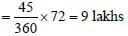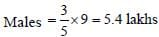Population of region S4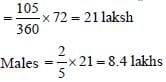Sum = 5.4 + 8.4 = 13.8 lakhs

SSC CGL Previous Year Questions: Data Interpretation - 6 - Question 7

DIRECTIONS: Population of five adjacent areas of a town, in the year of 2010, are represented in the following Piechart. the ratio of the numbers of males to that of females in these areas are stated in the table below. The total of the population in all the five areas is 72 lakh. Study the Pie-chart and the table and then answer the questions.Ratio of numbers of males (M) to females (F)       (SSC CGL 1st Sit. 2012)Q. The ratio of number of females in the area S2 to that in the area S5 is

Detailed Solution for SSC CGL Previous Year Questions: Data Interpretation - 6 - Question 7

Population of region S2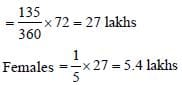Population of region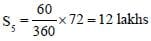Famales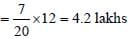Required ratio = 5.4 : 4.2 = 9 : 7

SSC CGL Previous Year Questions: Data Interpretation - 6 - Question 8

DIRECTIONS: Population of five adjacent areas of a town, in the year of 2010, are represented in the following Piechart. the ratio of the numbers of males to that of females in these areas are stated in the table below. The total of the population in all the five areas is 72 lakh. Study the Pie-chart and the table and then answer the questions.Ratio of numbers of males (M) to females (F)       (SSC CGL 1st Sit. 2012)Q. If, in the year 2010, there was an increase of 5% population in the area S1 and 8% increase in population of the area S3 compared to the previous year, then the ratio of population in the areas S1 and S3, in the year 2009 was

Detailed Solution for SSC CGL Previous Year Questions: Data Interpretation - 6 - Question 8

Population in 2009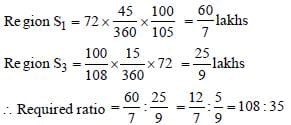SSC CGL Previous Year Questions: Data Interpretation - 6 - Question 9

DIRECTIONS: Population of five adjacent areas of a town, in the year of 2010, are represented in the following Piechart. the ratio of the numbers of males to that of females in these areas are stated in the table below. The total of the population in all the five areas is 72 lakh. Study the Pie-chart and the table and then answer the questions.Ratio of numbers of males (M) to females (F)       (SSC CGL 1st Sit. 2012)Q. The aver age of female population in all the five areas is lower than the female population in each of the areas

Detailed Solution for SSC CGL Previous Year Questions: Data Interpretation - 6 - Question 9

Number of females :
According to above explanation,
Region S1 ⇒ 9 – 5.4 = 3.6 lakhs
Region S2 ⇒ 5.4 lakhs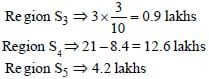Average =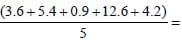5.34 lakhs

SSC CGL Previous Year Questions: Data Interpretation - 6 - Question 10

DIRECTIONS: The number of mobile simcards in 4 states are given in multiple bar diagrams. Study the diagram and answer the questions below.         (SSC CGL 2nd Sit.2012)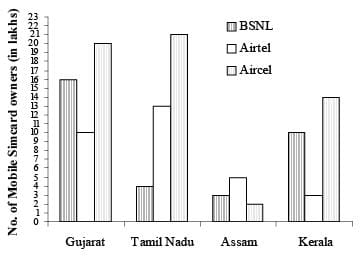Q. In Assam, the ratio of Air cell simcard and Airtel simcard sold is:

Detailed Solution for SSC CGL Previous Year Questions: Data Interpretation - 6 - Question 10

Required ratio = 2 : 5

SSC CGL Previous Year Questions: Data Interpretation - 6 - Question 11

DIRECTIONS: The number of mobile simcards in 4 states are given in multiple bar diagrams. Study the diagram and answer the questions below.         (SSC CGL 2nd Sit.2012)Q. In which state are there the largest number of owners of Airtel simcard?

Detailed Solution for SSC CGL Previous Year Questions: Data Interpretation - 6 - Question 11

It is obvious from the bar diagram.

SSC CGL Previous Year Questions: Data Interpretation - 6 - Question 12

DIRECTIONS: The number of mobile simcards in 4 states are given in multiple bar diagrams. Study the diagram and answer the questions below.         (SSC CGL 2nd Sit.2012)Q. Average of simcard sold in the four states in lakhs is

Detailed Solution for SSC CGL Previous Year Questions: Data Interpretation - 6 - Question 12

Required average = 121/4 = 30.25 lakhs

SSC CGL Previous Year Questions: Data Interpretation - 6 - Question 13

DIRECTIONS: The number of mobile simcards in 4 states are given in multiple bar diagrams. Study the diagram and answer the questions below.         (SSC CGL 2nd Sit.2012)Q. The range of BSNL simcard sold in the 4 states in lakhs is:

Detailed Solution for SSC CGL Previous Year Questions: Data Interpretation - 6 - Question 13

Required range = 16 – 3 = 13 lakhs

SSC CGL Previous Year Questions: Data Interpretation - 6 - Question 14

DIRECTIONS: The number of mobile simcards in 4 states are given in multiple bar diagrams. Study the diagram and answer the questions below.         (SSC CGL 2nd Sit.2012)Q. Of all the simcards sold in all the four states, the number of simcards sold in Gujarat is (approx)

Detailed Solution for SSC CGL Previous Year Questions: Data Interpretation - 6 - Question 14

Required percentage = (46/121) x 100 = 38.

SSC CGL Previous Year Questions: Data Interpretation - 6 - Question 15

DIRECTIONS: The graph shows Income and Expenditure of a company. Study the graph and answer the questions.        (SSC CGL 1st Sit.2012)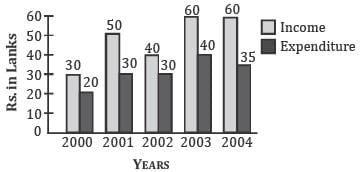Q. The number of years in which the income exceeds the average income is

Detailed Solution for SSC CGL Previous Year Questions: Data Interpretation - 6 - Question 15

Average income of company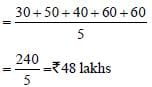The incomes of company in years 2001, 2003 and 2004 were greater than ₹48 lakhs.

SSC CGL Previous Year Questions: Data Interpretation - 6 - Question 16

DIRECTIONS: The graph shows Income and Expenditure of a company. Study the graph and answer the questions.        (SSC CGL 1st Sit.2012)Q. The difference in profit between 2001 and 2002 is

Detailed Solution for SSC CGL Previous Year Questions: Data Interpretation - 6 - Question 16

Required difference = 20 – 10 = ₹ 10 lakhs

SSC CGL Previous Year Questions: Data Interpretation - 6 - Question 17

DIRECTIONS: The graph shows Income and Expenditure of a company. Study the graph and answer the questions.        (SSC CGL 1st Sit.2012)Q. The profit was maximum in the year

Detailed Solution for SSC CGL Previous Year Questions: Data Interpretation - 6 - Question 17

Profit of company in 2004 = ₹ 25 lakhs

SSC CGL Previous Year Questions: Data Interpretation - 6 - Question 18

DIRECTIONS: The graph shows Income and Expenditure of a company. Study the graph and answer the questions.        (SSC CGL 1st Sit.2012)Q. The income in 2002 was equal to the expenditure in the year

Detailed Solution for SSC CGL Previous Year Questions: Data Interpretation - 6 - Question 18

Income of company in 2002
= ₹40 lakhs
Expenditure of company in 2003
= ₹40 lakhs

SSC CGL Previous Year Questions: Data Interpretation - 6 - Question 19

DIRECTIONS: The graph shows Income and Expenditure of a company. Study the graph and answer the questions.        (SSC CGL 1st Sit.2012)Q. The expenditure from 2002 to 2003 increased by

Detailed Solution for SSC CGL Previous Year Questions: Data Interpretation - 6 - Question 19

Required percentage increase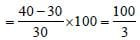= 33(1/3)%

SSC CGL Previous Year Questions: Data Interpretation - 6 - Question 20

DIRECTIONS: Study the graph and answer the questions that follows :
Circle graph given below shows the expenditure incurred in bringing out a book by a publisher.       (SSC CHSL 2012)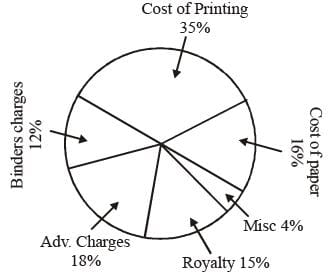Q. The central angle of the sector for the cost of the paper is :

Detailed Solution for SSC CGL Previous Year Questions: Data Interpretation - 6 - Question 20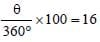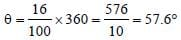SSC CGL Previous Year Questions: Data Interpretation - 6 - Question 21

DIRECTIONS: Study the graph and answer the questions that follows :
Circle graph given below shows the expenditure incurred in bringing out a book by a publisher.       (SSC CHSL 2012)Q. Royalty on the book is less than the Advertisement charges by:

Detailed Solution for SSC CGL Previous Year Questions: Data Interpretation - 6 - Question 21

Required difference (in % value)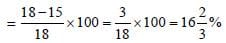SSC CGL Previous Year Questions: Data Interpretation - 6 - Question 22

DIRECTIONS: Study the graph and answer the questions that follows :
Circle graph given below shows the expenditure incurred in bringing out a book by a publisher.       (SSC CHSL 2012)Q. If 5500 copies are published, Miscellaneous expenditures amounts to ₹ 1848 and publisher’s profit is 25%, then marked price of each copy is :

Detailed Solution for SSC CGL Previous Year Questions: Data Interpretation - 6 - Question 22

Given,
Miscellaneous expenditure = ₹ 1848
⇒ 4% of the total expenditure cost for publishing 5500 copies = ₹ 1848
⇒ Total expenditure cost of 5500 copies (i.e. 100%)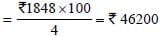⇒ Expenditure cost per copy =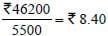So, marked price of each copy = ₹ 8.40 + 25% of 8.40
= 8.40 + 2.10
= ₹ 10.50

SSC CGL Previous Year Questions: Data Interpretation - 6 - Question 23

DIRECTIONS: Study the graph and answer the questions that follows :
Circle graph given below shows the expenditure incurred in bringing out a book by a publisher.       (SSC CHSL 2012)Q. If the cost of printing is ₹ 17,500, the Royalty is:

Detailed Solution for SSC CGL Previous Year Questions: Data Interpretation - 6 - Question 23

Cost of printing i.e., 35% = 17500
So, Royality i.e., 15% = (17500/35) x 15 = 7500

SSC CGL Previous Year Questions: Data Interpretation - 6 - Question 24

DIRECTIONS: Study the graph and answer the questions that follows :
Circle graph given below shows the expenditure incurred in bringing out a book by a publisher.       (SSC CHSL 2012)Q. If the Miscellaneous charges is ₹ 6,000, the Advertisement charges are:

Detailed Solution for SSC CGL Previous Year Questions: Data Interpretation - 6 - Question 24

Miscellaneous charges i.e. 4% = 6000
= (6000/4) x 18 = 27000

SSC CGL Previous Year Questions: Data Interpretation - 6 - Question 25

DIRECTIONS: Read the following chart and answer the questions that follows :
The following pie-chart shows the preference of musical instruments of 60,000 people surveyed over whole India.       (SSC CHSL 2012)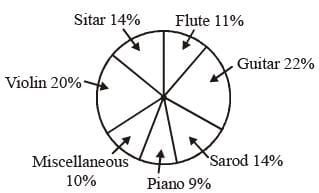Q. The number of people who prefer Guitar is greater than the total number of people who prefer either Flute or Piano by :

Detailed Solution for SSC CGL Previous Year Questions: Data Interpretation - 6 - Question 25

No. of people who prefer guitar = 22% of 60000 = 13200
No. of people who prefer Flute or Piano = (11 + 9)% of 60000 = 12000
Required difference = 13200 – 12000 = 1200.

SSC CGL Previous Year Questions: Data Interpretation - 6 - Question 26

DIRECTIONS: Read the following chart and answer the questions that follows :
The following pie-chart shows the preference of musical instruments of 60,000 people surveyed over whole India.       (SSC CHSL 2012)Q. If 16(2/3)% of the people who prefer Piano, would go with the people who prefers Flute, the percentage of people who prefer Flute would have been :

Detailed Solution for SSC CGL Previous Year Questions: Data Interpretation - 6 - Question 26

No. of people who prefer piano = 9% of 60000 = 5400
According to question, 16(2/3)% no. of the people who prefer piano would go with flute.
Therefore, (50/3)% of 5400 = 900
Hence, the required percentage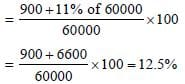SSC CGL Previous Year Questions: Data Interpretation - 6 - Question 27

DIRECTIONS: Read the following chart and answer the questions that follows :
The following pie-chart shows the preference of musical instruments of 60,000 people surveyed over whole India.       (SSC CHSL 2012)Q. The number of people who prefer the musical instrument Sarod is :

Detailed Solution for SSC CGL Previous Year Questions: Data Interpretation - 6 - Question 27

Required number = 14% of 60000 = (14/100) x 60000 = 8400

SSC CGL Previous Year Questions: Data Interpretation - 6 - Question 28

DIRECTIONS: Read the following chart and answer the questions that follows :
The following pie-chart shows the preference of musical instruments of 60,000 people surveyed over whole India.       (SSC CHSL 2012)Q. The total number of people who prefer either Sarod or Guitar, is greater than the total number of people who prefer either Violin or Sitar by :

Detailed Solution for SSC CGL Previous Year Questions: Data Interpretation - 6 - Question 28

Total number of people who prefer either Sarod or Guitar
= 14% of 60000 + 22% of 60000
⇒ 8400 + 13200 = 21600
Total number of people of who prefer violin or Sitar
= 20% of 60000 + 14% of 60000
⇒ 12000 + 8400 = 20400
Required difference = 21600 – 20400 = 1200

SSC CGL Previous Year Questions: Data Interpretation - 6 - Question 29

DIRECTIONS: Read the following chart and answer the questions that follows :
The following pie-chart shows the preference of musical instruments of 60,000 people surveyed over whole India.       (SSC CHSL 2012)Q. If 2100 people be less from the number of people who prefer Flute, the percentage of people who prefer Flute would have been:

Detailed Solution for SSC CGL Previous Year Questions: Data Interpretation - 6 - Question 29

No. of people who prefer flute = 11% of 60,000
= (11/100) x 60000 = 6600
2100 people be less from the people who prefer flute.
Therefore, 6600 – 2100 = 4500
Required percentage = (4500/60000) x 100 = 7.5%

SSC CGL Previous Year Questions: Data Interpretation - 6 - Question 30

DIRECTIONS: The pie-chart given below shows the distribution of workforce by occupational category for country X in 1981 and 1995. Study the chart and answer the questions no. 15 to 19.        (SSC Sub. Ins. 2012)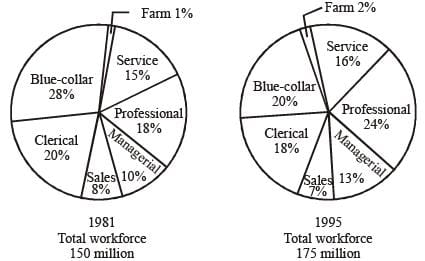Q. The percentage decrease in the number of Blue-Collar workers in the workforce of country X from 1981 to 1995 is

Detailed Solution for SSC CGL Previous Year Questions: Data Interpretation - 6 - Question 30

% of Blue collar workers in 1981 = 28% of 150 = 42
% of Blue collar workers in 1995
= 20% of 175 = 35
% decrease =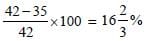## SSC CGL (Tier - 1) - Previous Year Papers (Topic Wise)

250 tests
Information about SSC CGL Previous Year Questions: Data Interpretation - 6 Page
In this test you can find the Exam questions for SSC CGL Previous Year Questions: Data Interpretation - 6 solved & explained in the simplest way possible. Besides giving Questions and answers for SSC CGL Previous Year Questions: Data Interpretation - 6, EduRev gives you an ample number of Online tests for practice

250 tests